Jim Dehner

Tableau How to’s,  Use Cases, and Forums Questions

Hey #DataFam

Let’s Talk

Open for

## Cannot Mix Aggregate and Non-aggregate

One of the most frequent questions we see on the Forum results from trying mixing aggregate and non-aggregates in the same calculation – But why is that a problem and how do you resolve it –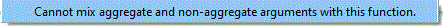Next time you load a data file into Tableau, open the data source tab and spend a moment to look at the data structure.  This might look like a spreadsheetNow, open a worksheet and make a calculation to determine the Profit Percent as Profit / Sales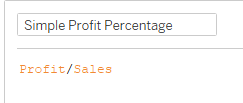Return to the data source tab and a Column (Measure) has been added to the table with the results of the calculation for each "Row" of data –
It's just what we'd expect from a spreadsheet calculator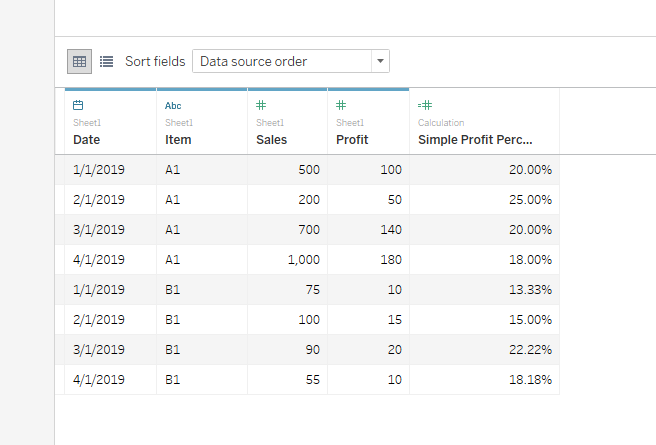Go to the worksheet and make a viz with totals and subtotals.  What's going on?
The row profit percentages are correct but the totals and subtotals are wrongTableau aggregates measures as they are brought to the viz so the individual profit percentages are summed in the viz – the problem started with the calculation – Sales and Profit need to be aggregated in the calculation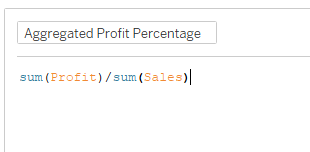Now on the Data Source tab, the Aggregate Profit Percent look just like the Simple valuesBut when we add the new measure to the viz we get the correct totals and subtotals
Note also the measure is brought to the viz as and (AGG)regate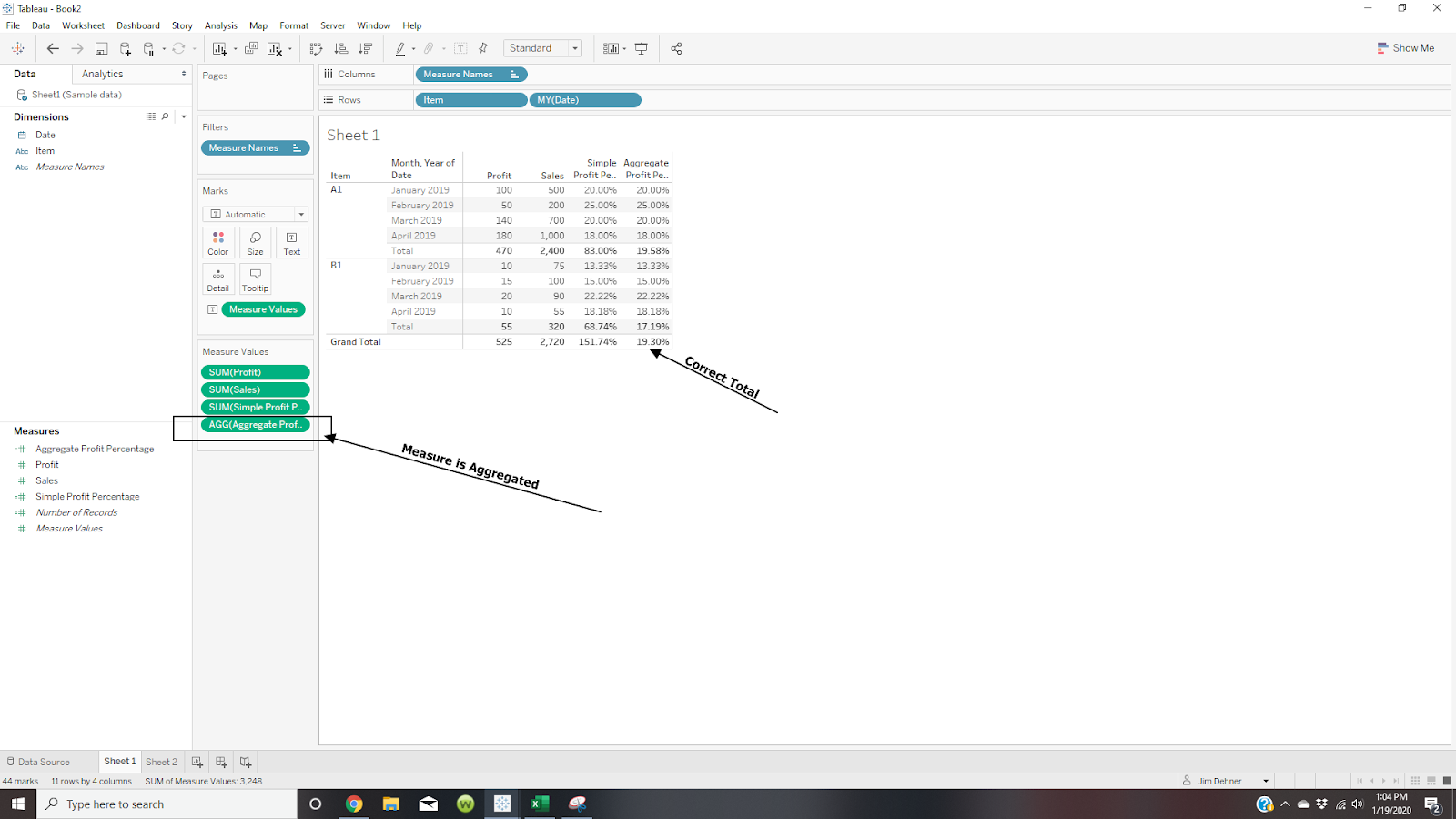So you can see how using aggregation in a calculation will affect the result but it is also the source of the aggregate – non-aggregate problem.The message simply means that if one dimension or measure is aggregated in a calculation then ALL the measures and dimensions in the calculation must be aggregated– But sometimes it is not easy to see which dimension or measures need to be aggregated.  See the following examples:

1-Table Calculations

Table calculations are aggregations so other measures in the calculation need to be aggregated too – here Difference is a table calculation so Sales needs to be aggregated :Aggregating Sales with Sum() solves the problem2-Embedded Dates

Often dates are embedded in a calculation that includes an aggregation –Here Sales in aggregated so Order Date and Ship Date need to be aggregated – Yes aggregate Dates – sure Dates and string (text) dimension can be aggregated with Attr(), Min() or Max() – your choice will depend on the analysis you are doing

This is just one way around the problem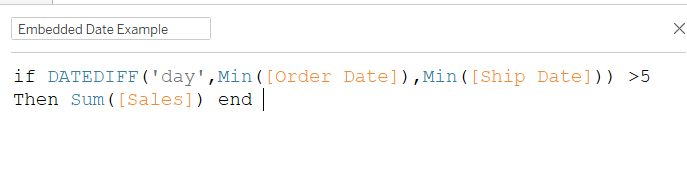Note: removing the sum() from sales would also work

3 Aggregations caused by LOD's

Sometimes, dimensions within a LOD cause the problem – The argument in a LOD must be aggregated – here Sales is aggregated but Category is notThere are 2 solutions – the first is to use a Min() or Max to aggregate the Category (Note:  Attr() can not be used in a LOD)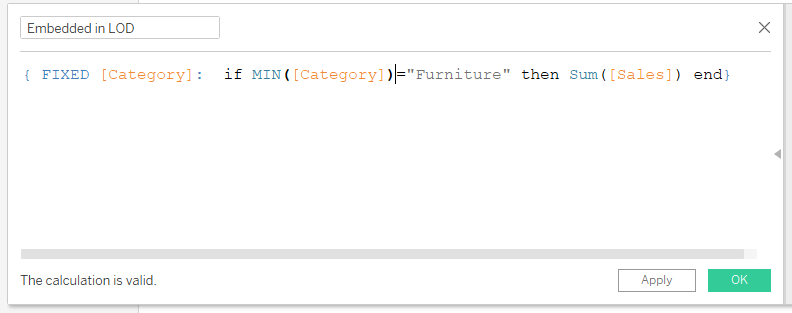The second is to move the Sum() outside the conditional statement in the LOD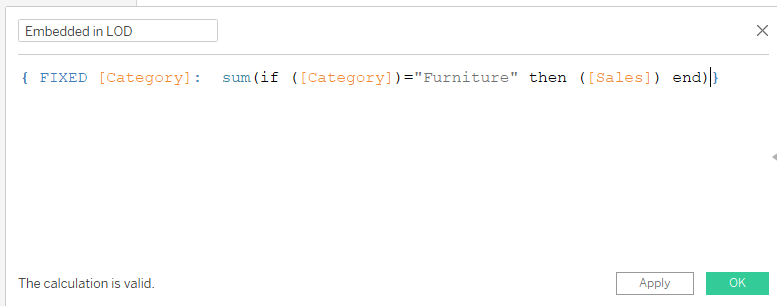Either solution will work

4 Using a LOD to resolve an aggregation problem

This example calculates the COGS percent to Sales
determine  COGS: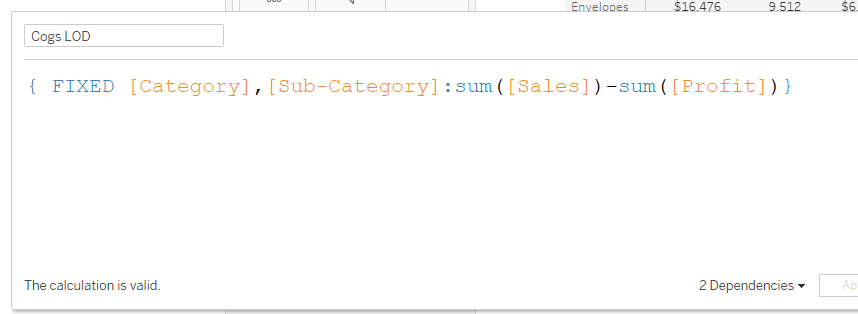But it results in an error when used to calculate the percent to SalesLODs create a virtual layer in your data set that is at a different level than the data itself BUT they are not an aggregate.   To correct the error aggregate the LOD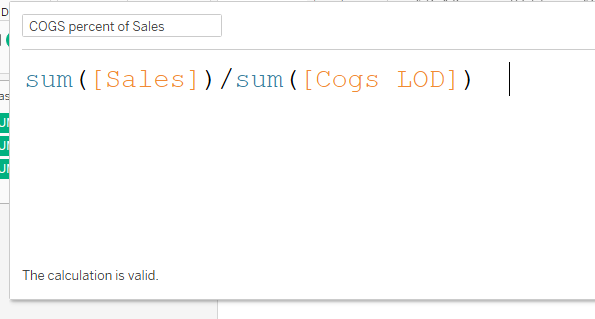5 Value at Max Date

A common question is to find the value on the last date – there are 2 ways – My preferred is using a LOD to find the last date in the data for each sub-categoryThen the sales value on that date isBut it returns an error because the Sales are aggregated but neither the order date nor the LOD is – so a solution would be to aggregate the date and the LOD – here I used Min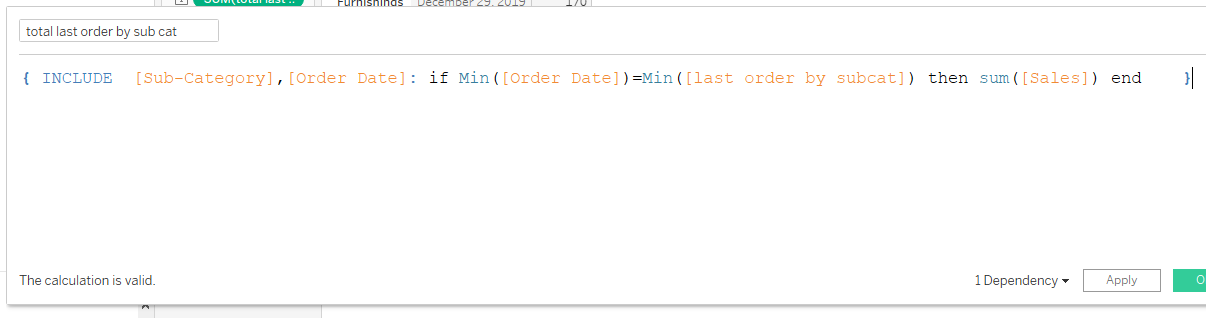An alternative would be to use a table calculation (here Last()) but it also returns an error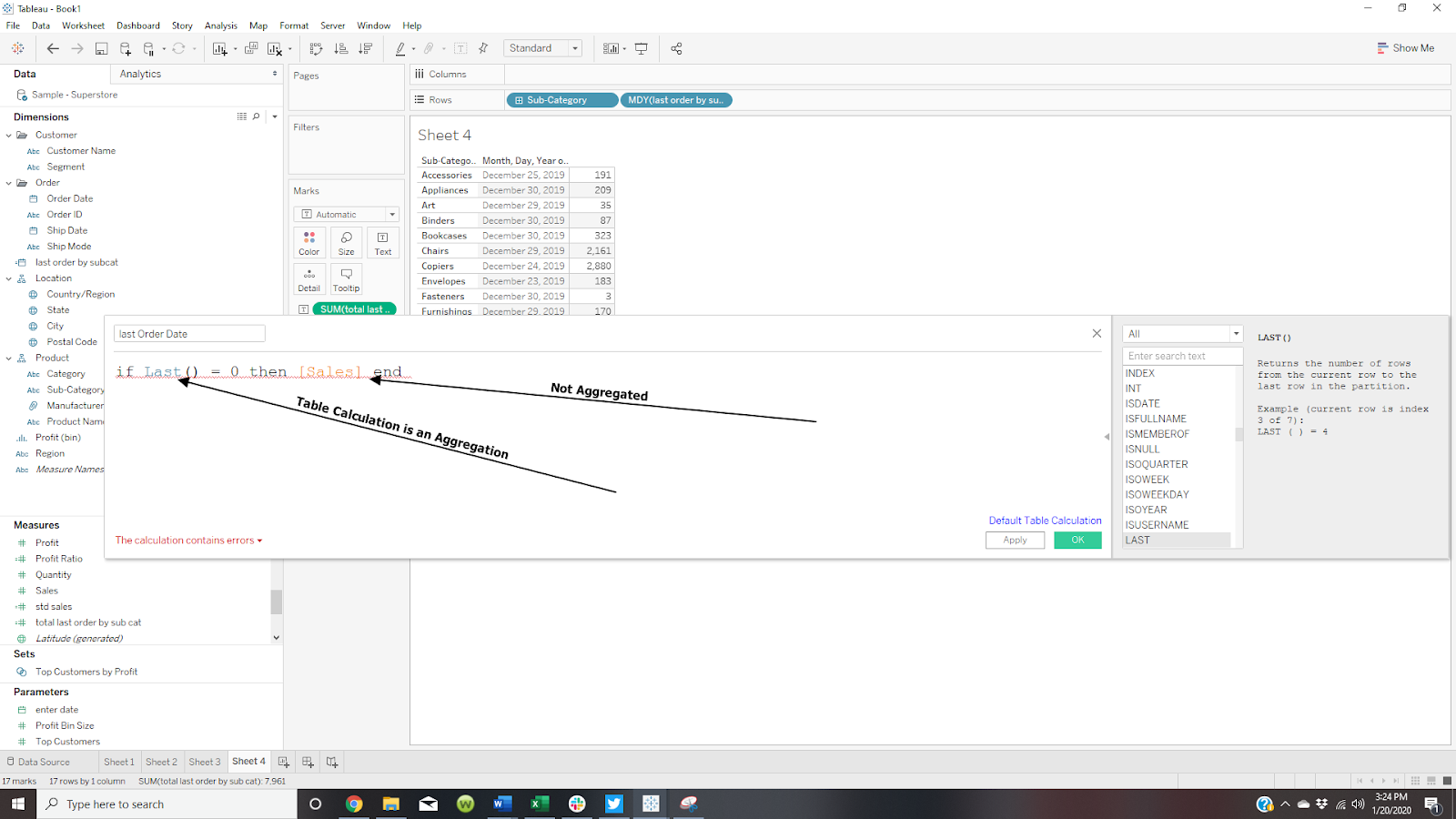This can be resolved by aggregating SalesThe process to Identify the Aggregate

There could be many more examples and still not get to the one you has you stumped – Fortunately, there is an easy way to know which of the dimensions or measure in your calculation are aggregated and which are not –

• Open the calculation and drag the measures to the Marks card –
• IF they show up with an AGG() then they are already aggregated –
• If Tableau tried to aggregate them with SUM()  then they are not aggregated and you will need to decide which aggregation best fits your analysis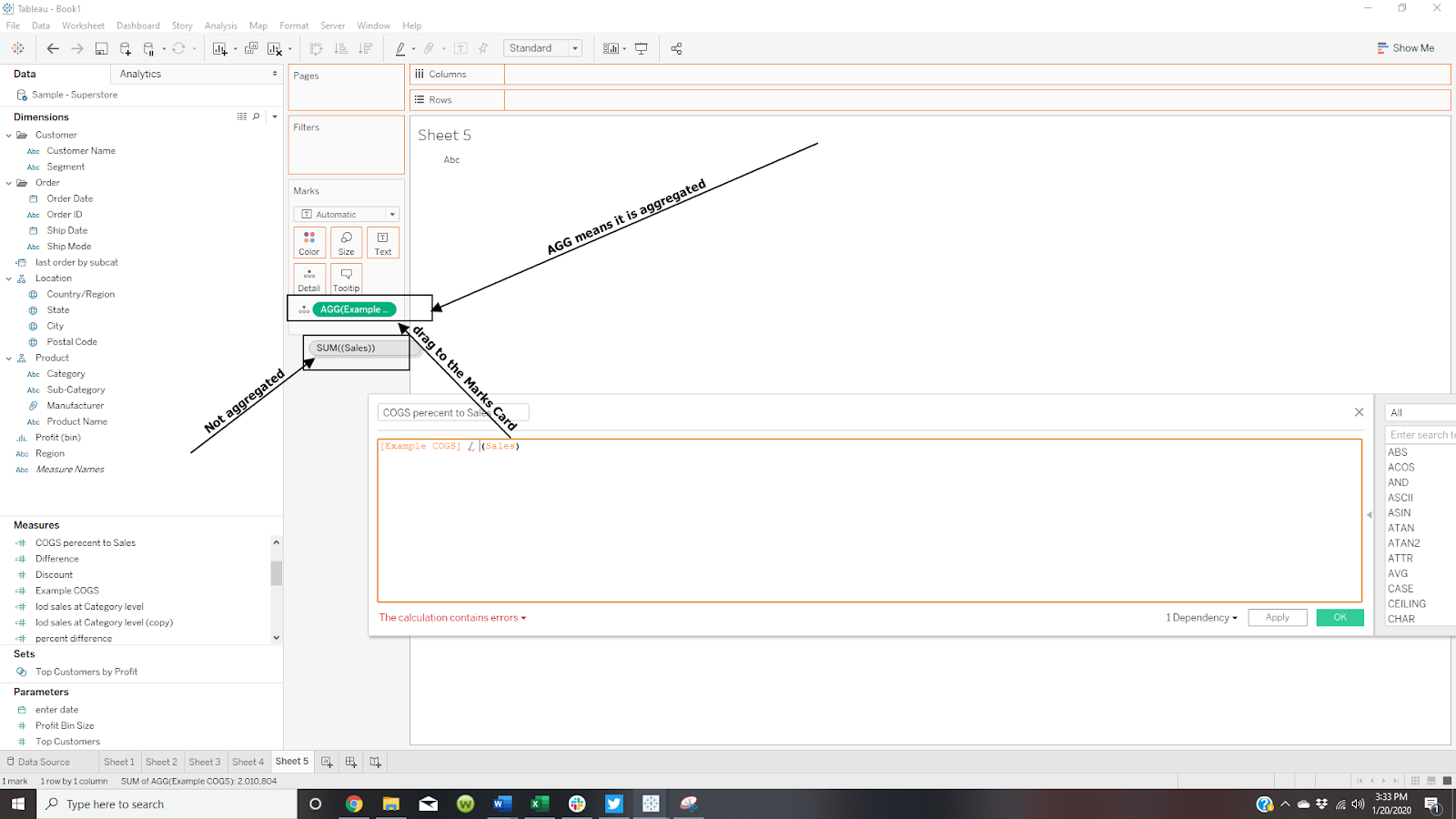Hope this helps – If you have specific examples where you need some help feel free to ping me here or add a post to the Forum

Enjoy
Jim

## Top Posts

### 7 Responses

1.anncutrell says:

Great resource for teaching. Thanks Jim!

2.www.jimdehner.blogspot.com says:

Thanks Ann – I'm doing a series on FAQ's from the Forum – stop back – the next is on Nulls

3.Krishna says:

Thanks for sharing Jim. It really helpful.

4.Tim Beard says:

Excellent post Jim – thank you!
I understand the issue, but it still catches me. Your tips to identify what are aggregates or not were particularly useful.
I recently voted on this idea to visually differentiate between aggregates and non-aggregates: https://community.tableau.com/ideas/10853

5.Unknown says:

Hi Jim,
I wonder if you can help me understand and resolve a problem I am struggling with. I have about 10 years of data joined by date at the day level. For three different columns (say A, B and C) I have the a value that corresponds to the value on that date.

I have a bar chart display that has the date grouped by year and I want to show the "total" value from each of A,B and C that corresponds with the last day of that year where I have a date. So – for 2011 it might be 30-Dec-11 as that is the last day I have a value in that year. I know this because I have created MAX([Date]) and filtered by that date.

I simplistically thought I would be able to grab the corresponding value from A, B or C that exists in the column for the MAX(Date) at the year level, however, what I get is SUM([A] etc. ) which is the sum of all the values in A up until the last day of the year. A huge number!

I've tried, LOOKUP with INDEX and LAST etc. in a calculated field using MAX([Date])but am blocked in grabbing this atomic value from the column as it requires an aggregated value (SUM([Total])). I can resolve this, pretty sure, by creating a new column in my data source that shows only the additions or subtractions in A, B or C and not their "current" value for that date. In this case I think the SUM([A]) will correspond, but I figure there is more likely something I am fundamentally missing to implement this with the data structured as is.

Is my explanation clear? and if you have a moment, do you see something obvious you could point out to me?

6.www.jimdehner.blogspot.com says:

Hi
Thanks great question – just reading the post it sounds like the data need to be pivoted – but without seeing the actual workbook it is a little difficult to provide a specific solution- Suggest you post your question on the Forum at https://community.tableau.com/community/forums and include your TWBX workbook –
Thanks

7.Tim Beard says:

This comment has been removed by the author.